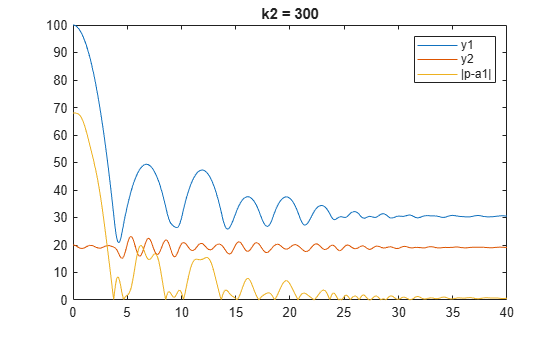# LPV Model of Bouncing Ball

This example shows how to construct a linear parameter-varying (LPV) representation of a system that exhibits multi-mode dynamics using `lpvss`.

The function `BouncingBallDF` defines the dynamics of this system. For more information on this model, see Using LTI Arrays for Simulating Multi-Mode Dynamics.

Define uncompressed spring lengths `a1` and `a2` and initial mass heights `h1` and `h2`.

```a1 = 12; % uncompressed length of spring 1 (mm) a2 = 20; % uncompressed length of spring 2 (mm) h1 = 100; % initial height of mass m1 (mm) h2 = a2; % initial height of mass m2 (mm)```

### Moderate Floor Stiffness

First simulate the response for a soft surface by setting the spring constant for the second mass `k2` to 300 g/s^2. The state is $\mathit{x}=\left[{\mathit{y}}_{1},{\stackrel{˙}{\mathit{y}}}_{1},{\mathit{y}}_{2},{\stackrel{˙}{\mathit{y}}}_{2}\right]$.

The input is the constant $\mathit{u}$ = 1 (the forcing term is $\mathit{F}=\mathit{mgu}$).

```k2 = 300; % spring constant for second mass (g/s^2) sys = lpvss("ygap",@(t,p) BouncingBallDF(t,p,k2)); % p = ygap = y1-y2 t = 0:0.05:40; pFcn = @(t,x,u) x(1)-x(3); xinit = [h1;0;h2;0]; [y,~,~,~,p] = step(sys,t,pFcn,RespConfig('InitialState',xinit)); figure(1), plot(t,y(:,1),t,y(:,2),t,abs(p-a1)) legend('y1','y2','|p-a1|') title('k2 = 300')```The curves show the position of the masses. At the start of simulation, mass 1 undergoes free fall until it hits mass 2. The collision causes the mass 2 to be displaced, but it recoils quickly and bounces mass 1 back. The two masses are in contact for the time duration where ${\mathit{y}}_{\mathrm{gap}}<\mathrm{a1}$. When the masses settle down, their equilibrium values are determined by the static settling due to gravity.

### High Floor Stiffness

Now simulate the response for a hard surface by setting `k2` to 30,000 g/s^2.

```k2 = 3e4; % spring constant for second mass (g/s^2) sys = lpvss("ygap",@(t,p) BouncingBallDF(t,p,k2)); t = 0:0.05:80; [y,~,~,~,p] = step(sys,t,pFcn,RespConfig('InitialState',xinit)); figure(2), plot(t,y(:,1),t,y(:,2),t,abs(p-a1)) legend('y1','y2','|p-a1|') title('k2 = 3e4')```For high floor stiffness, the collision does not cause the mass 2 to be displaced. It instead bounces the mass 1 back to a higher position; also, it takes more bounces for mass 1 to settle.

### Data Function Code

`type BouncingBallDF.m`
```function [A,B,C,D,E,dx0,x0,u0,y0,Delays] = BouncingBallDF(~,p,k2) % p = gap y1-y2 m1 = 7; % first mass (g) k1 = 100; % spring constant for first mass (g/s^2) c1 = 2; % damping coefficient associated with first mass (g/s) m2 = 20; % second mass (g) %k2 = 300; % spring constant for second mass (g/s^2) c2 = 5; % damping coefficient associated with second mass (g/s) g = 9.81; % gravitational acceleration (m/s^2) a1 = 12; % uncompressed lengths of spring 1 (mm) a2 = 20; % uncompressed lengths of spring 2 (mm) if p>a1 % Uncoupled A11 = [0 1; 0 0]; B11 = [0; -g]; C11 = [1 0]; D11 = 0; A12 = [0 1; -k2/m2, -c2/m2]; B12 = [0; -g+(k2*a2/m2)]; C12 = [1 0]; D12 = 0; A = blkdiag(A11, A12); B = [B11; B12]; C = blkdiag(C11, C12); D = [D11; D12]; else A = [ 0 1, 0, 0; ... -k1/m1, -c1/m1, k1/m1, c1/m1;... 0, 0, 0, 1; ... k1/m2, c1/m2, -(k1+k2)/m2, -(c1+c2)/m2]; B = [0; -g+k1*a1/m1; 0; -g+(k2/m2*a2)-(k1/m2*a1)]; C = [1 0 0 0; 0 0 1 0]; D = [0;0]; end E = []; dx0 = []; x0 = []; u0 = []; y0 = []; Delays = []; ```